# 你的颜值能打多少分？让飞桨来告诉你

2020/01/09 18:1501

VGGNet介绍

VGGNet 由牛津大学的视觉几何组(Visual Geometry Group)和 Google DeepMind 公司提出，是 ILSVRC-2014 中定位任务第一名和分类任务第二名。提出 VGGNet 的主要目的是为了探究在大规模图像识别任务中，卷积网络深度对模型精确度的影响。通过VGGNet，研究人员证明了基于尺寸较小的的卷积核，增加网络深度可以有效提升模型的效果。VGGNet结构简单，模型的泛化能力好，因此受到研究人员青睐而广泛使用，到现在依然经常被用作图像特征提取。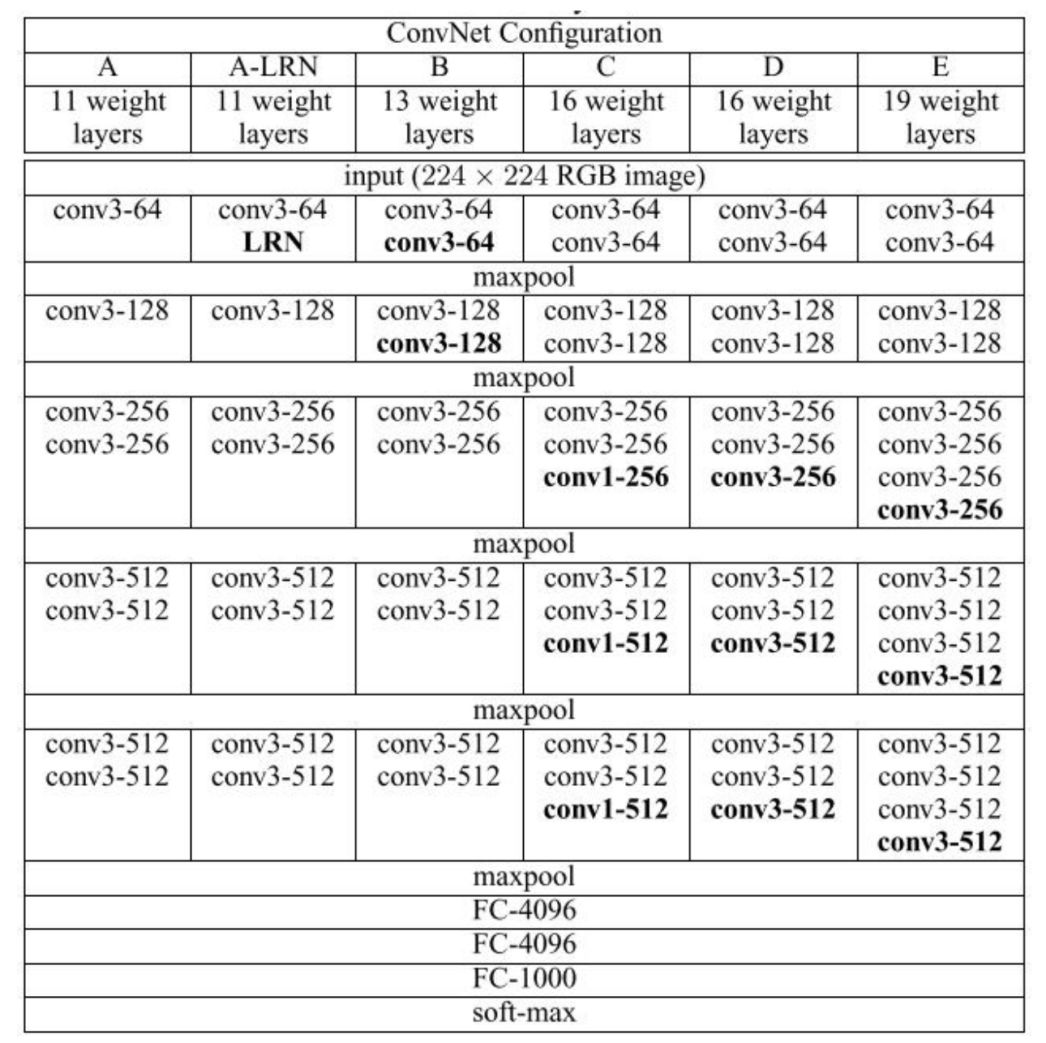VGGNet引入“模块化”的设计思想，将不同的层进行简单的组合构成网络模块，再用模块来组装完整网络，而不再是以“层”为单元组装网络。VGGNet有5种不同的VGGNet 配置，如上表所示。其中每一列代表一种网络配置，分别用 A~E 来表示。从表格中可以看出所有VGG配置都有五个卷积模块，模块中所有卷积都是3×3卷积核(conv3)，因此特征图的尺寸在模块内不是变的，每个模块卷积之后紧接着最大池化层。最浅的A网络也称为VGG-11，它包含11个带可学习参数层，最深的E网络也称为VGG-19，包含19个带可学习参数层。业界普遍认为，更深的网络具有比浅网络更强的表达能力，更能刻画现实，完成更复杂的任务，VGG-19结构如下图所示。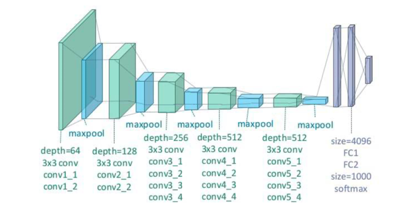VGGNet是基于 AlexNet网络的，VGG在Alexnet（注：Alexnet介绍参考《深度学习导论与应用实践》5.7.2节内容）基础上对深度神经网络在深度和宽度上做了更多深入的研究，相较于Alexnet而言，VGG中的3×3卷积核的感受野要小，但是两个3×3 卷积的卷积级联其感受野相当于5×5卷积，三个3×3卷积级联感受野相当于7×7卷积，为什么要这样做呢？

02

### 注：原始数据集中每张图像并没有专门对人脸部分进行剪裁，图像中包含大量背景。因此，我们将提前去除了非人脸的部分并将图片大小剪裁为224x224，存储为本次实践中使用的数据集，如下图所示，图片命名格式是：颜值得分-图片编号。处理好的数据集获取地址为 https://pan.baidu.com/s/1B9Hlo031BABA6Hj-r16EQw。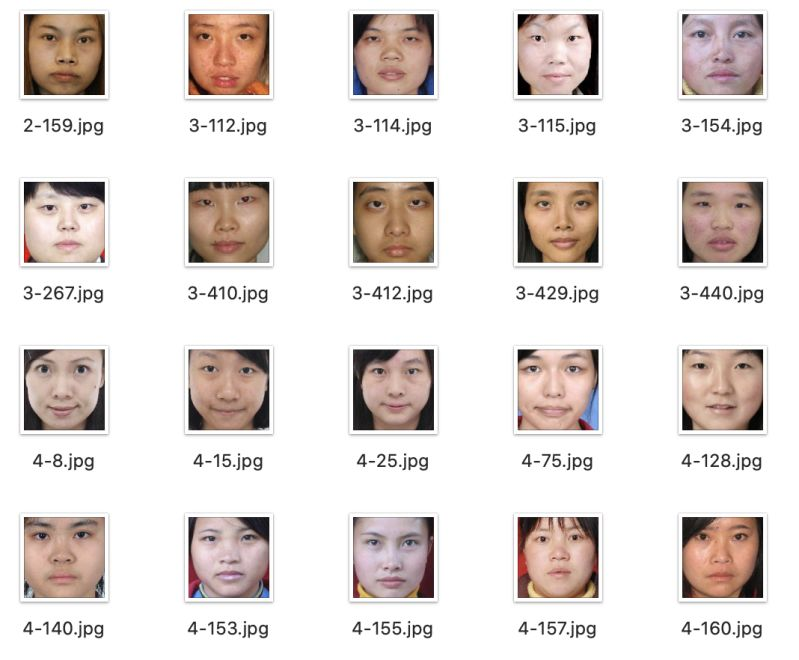### 注意：由于飞桨中交叉熵损失函数要求label必须从0开始，而数据集label从1开始，所以在获取label时，我们将label减1。

#导入必要的包
import os
import numpy as np
from PIL importImage
frommultiprocessing import cpu_count
importmatplotlib.pyplot as plt

def data_mapper(data):
img, label = data
#将img数组进行进行归一化处理，得到0到1之间的数值
img= img.flatten().astype('float32')/255.0
return img, int(label)

print(data_path)
for image in os.listdir(data_path):
label = int(image.split('-'))-1       #label减1
img = os.path.join(data_path+ '/' +image)
yield img, label


#构造训练、测试数据提供器
BATCH_SIZE = 16
train_r =data_r(data_path='/home/aistudio/face_image_train')
batch_size=BATCH_SIZE)
test_r=data_r(data_path='/home/aistudio/face_image_test')


03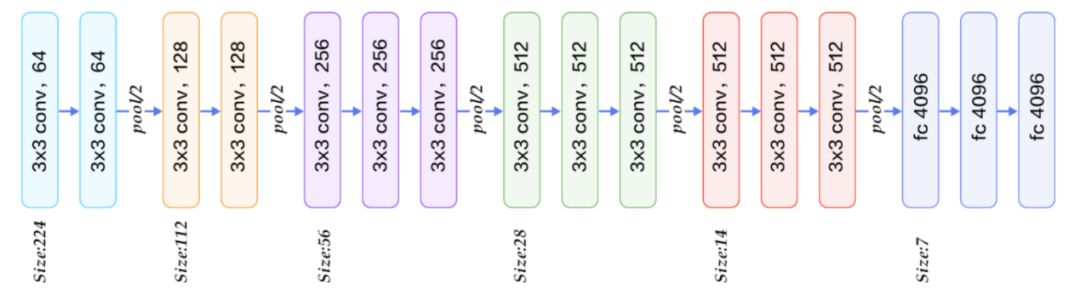def vgg_bn_drop(image, type_size):
def conv_block(ipt, num_filter, groups,dropouts):
return fluid.nets.img_conv_group(
input=ipt, # 具有[N，C，H，W]格式的输入图像
pool_size=2,
pool_stride=2,
conv_num_filter=[num_filter] *groups, # 过滤器个数
conv_filter_size=3, # 过滤器大小
conv_act='relu',
conv_with_batchnorm=True, # 表示在 Conv2d Layer 之后是否使用 BatchNorm
conv_batchnorm_drop_rate=dropouts,#表示 BatchNorm 之后的 Dropout Layer 的丢弃概率
pool_type='max') # 最大池化

conv1 = conv_block(image, 64, 2, [0.0, 0])
conv2 = conv_block(conv1, 128, 2, [0.0, 0])
conv3 = conv_block(conv2, 256, 3, [0.0,0.0, 0])
conv4 = conv_block(conv3, 512, 3, [0.0,0.0, 0])
conv5 = conv_block(conv4, 512, 3, [0.0,0.0, 0])

drop = fluid.layers.dropout(x=conv2,dropout_prob=0.5)
fc1 = fluid.layers.fc(input=drop, size=512,act=None)
bn = fluid.layers.batch_norm(input=fc1, act='relu')
drop2 = fluid.layers.dropout(x=bn,dropout_prob=0.5)
fc2 = fluid.layers.fc(input=drop2, size=1024,act=None)
predict = fluid.layers.fc(input=fc2,size=type_size, act='softmax')
return predict


# 定义输入输出层
# 定义两个张量
image =fluid.layers.data(name='image', shape=[3, 224, 224], dtype='float32')
label =fluid.layers.data(name='label', shape=, dtype='int64')


# 获取分类器
predict=vgg_bn_drop(image,5)


# 定义损失函数和准确率函数
cost =fluid.layers.cross_entropy(input=predict, label=label)
avg_cost =fluid.layers.mean(cost)
accuracy =fluid.layers.accuracy(input=predict, label=label)


# 克隆main_program得到test_program，使用参数for_test来区分该程序是用来训练还是用来测试#注意：该fluid.default_main_program().clone()请在optimization之前使用.test_program =fluid.default_main_program().clone(for_test=True)接着定义优化算法，这里使用的是Adam优化算法，指定学习率为0.002。
# 定义优化方法
opts = optimizer.minimize(avg_cost)


（1）fluid.default_startup_program：定义了创建模型参数，输入输出，以及模型中可学习参数的初始化等各种操作，由框架自动生成，使用时无需显示地创建；

（2）fluid.default_main_program ：定义了神经网络模型，前向反向计算，以及优化算法对网络中可学习参数的更新，使用Fluid的核心就是构建起 default_main_program。

04

#定义使用CPU还是GPU，使用CPU时use_cuda = False,使用GPU时use_cuda = True
use_cuda = False
place = fluid.CUDAPlace(0) if use_cuda else fluid.CPUPlace()
#创建一个Executor实例exe
exe =fluid.Executor(place)
#正式进行网络训练前，需先执行参数初始化
exe.run(fluid.default_startup_program())


feeder = fluid.DataFeeder(place=place, feed_list=[image, label])


EPOCH_NUM = 60
#训练过程数据记录
all_train_iter=0
all_train_iters=[]
all_train_costs=[]
all_train_accs=[]

#测试过程数据记录
all_test_iter=0
all_test_iters=[]
all_test_costs=[]
all_test_accs=[]
model_save_dir ="/home/aistudio/work"

for pass_id inrange(EPOCH_NUM):
# 开始训练
for batch_id, data inenumerate(train_reader()):      #遍历训练集，并为数据加上索引batch_id
train_cost,train_acc =exe.run(program=fluid.default_main_program(),#运行主程序
feed=feeder.feed(data),                 #喂入一个batch的数据
fetch_list=[avg_cost, acc])         #fetch均方误差和准确率
all_train_iter=all_train_iter+BATCH_SIZE
all_train_iters.append(all_train_iter)
all_train_costs.append(train_cost)
all_train_accs.append(train_acc)
#每10次batch打印一次训练、进行一次测试
if batch_id % 10== 0:
print('Pass:%d, Batch:%d,Cost:%0.5f, Accuracy:%0.5f' %
(pass_id, batch_id, train_cost,train_acc))
# 开始测试
test_costs = []                                                        #测试的损失值
test_accs = []                                                         #测试的准确率
for batch_id, data in enumerate(test_reader()):
test_cost, test_acc =exe.run(program=test_program,              #执行训练程序
feed=feeder.feed(data),            #喂入数据
fetch_list=[avg_cost, acc])         #fetch 误差、准确率
test_costs.append(test_cost)                                #记录每个batch的误差
test_accs.append(test_acc)                             #记录每个batch的准确率
all_test_iter=all_test_iter+BATCH_SIZE
all_test_iters.append(all_test_iter)
all_test_costs.append(test_cost)
all_test_accs.append(test_acc)
# 求测试结果的平均值
test_cost = (sum(test_costs) /len(test_costs))        #计算误差平均值（误差和/误差的个数）
test_acc = (sum(test_accs) /len(test_accs))  #计算准确率平均值（ 准确率的和/准确率的个数）
print('Test:%d, Cost:%0.5f, ACC:%0.5f' %(pass_id, test_cost, test_acc))

# 保存模型
# 如果保存路径不存在就创建
if not os.path.exists(model_save_dir):
os.makedirs(model_save_dir)
print('savemodels to %s' % (model_save_dir))
fluid.io.save_inference_model(model_save_dir,  # 保存预测Program的路径
['image'],      #预测需要feed的数据
[predict],       #保存预测结果
exe)             #executor 保存预测模型


05

def draw_cost_process(title,iters,costs,label_cost):
plt.title(title, fontsize=24)
plt.xlabel("iter", fontsize=20)
plt.ylabel("cost", fontsize=20)
plt.plot(iters,costs,color='red',label=label_cost)
plt.legend()
plt.grid()
plt.show()
defdraw_acc_process(title,iters,acc,label_acc):
plt.title(title, fontsize=24)
plt.xlabel("iter", fontsize=20)
plt.ylabel("acc", fontsize=20)
plt.plot(iters,acc,color='green',label=label_acc)
plt.legend()
plt.grid()
plt.show()


#调用绘制曲线
draw_acc_process("training",all_train_iters, all_train_accs, "trainning acc")
draw_acc_process("testing",all_test_iters, all_test_accs, "test acc")
draw_cost_process("training",all_train_iters, all_train_costs, "trainning acc")
draw_cost_process("testing",all_test_iters, all_test_costs, "test acc")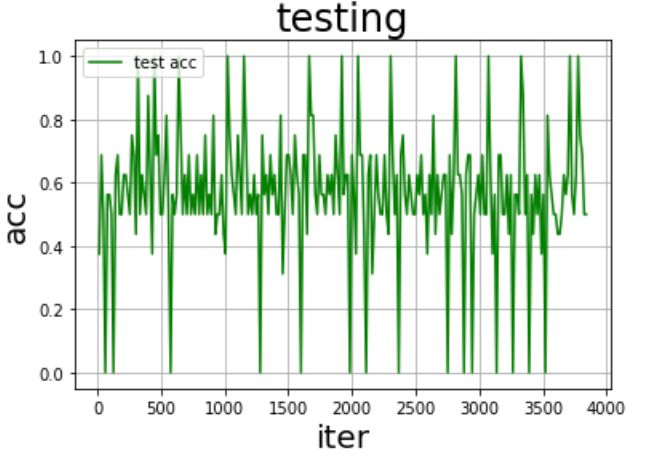06

#图片预处理
im = Image.open(file)
im =im.resize((224, 224), Image.ANTIALIAS)                  #resize 图像大小为224*224
if np.array(im).shape == 4             #判断图像通道数，若为4通道，则将其转化为3通道
im = np.array(im)[:,:,:3]
im = np.array(im).reshape(1, 3, 224,224).astype(np.float32) #返回新形状的数组,把它变成一个 numpy 数组以匹配数据馈送格式。
im = im / 255.0                                             #归一化到[-1~1]之间
return im


# 加载模型并开始预测
infer_exe =fluid.Executor(place)
infer_img='/home/aistudio/image1.jpg'
#获取训练好的模型
#从指定目录中加载 推理model(inference model)
[inference_program,#预测用的program
feed_target_names,#是一个str列表，它包含需要在推理 Program 中提供数据的变量的名称。
fetch_targets] = fluid.io.load_inference_model(model_save_dir,infer_exe)#fetch_targets：是一个 Variable 列表，从中我们可以得到推断结果。

img =Image.open(infer_img)
plt.imshow(img)   #根据数组绘制图像
plt.show()        #显示图像

# 开始预测
results =infer_exe.run(
inference_program,                      #运行预测程序
feed={feed_target_names: image},#喂入要预测的数据
fetch_list=fetch_targets)              #得到推测结果


# 获取概率最大的label
print('results',results)
label_list =["1", "2", "3", "4", "5"]
print("inferresults: %s" % label_list[np.argmax(results)])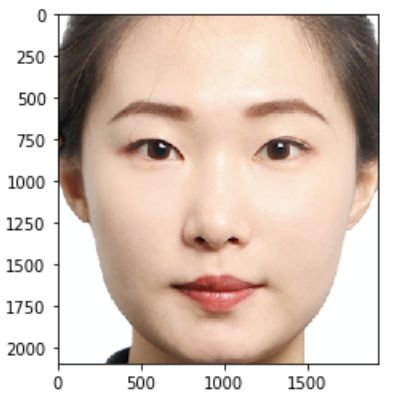results[array([[1.7734790e-12, 8.6307369e-02, 1.1037191e-03, 9.1258878e-01,
7.8247488e-08]], dtype=float32)]infer results: 4


https://aistudio.baidu.com/aistudio/course/introduce/998### 作者的其它热门文章

0
0 收藏

0 评论
0 收藏
0